# Polymer – Part 3 (Structured Questions)

Question 4:
Diagram 1.1 shows an experiment to study the effect of vinegar and ammonium solution on latex.
Diagram 1.2 shows the result of the experiment after 30 minutes.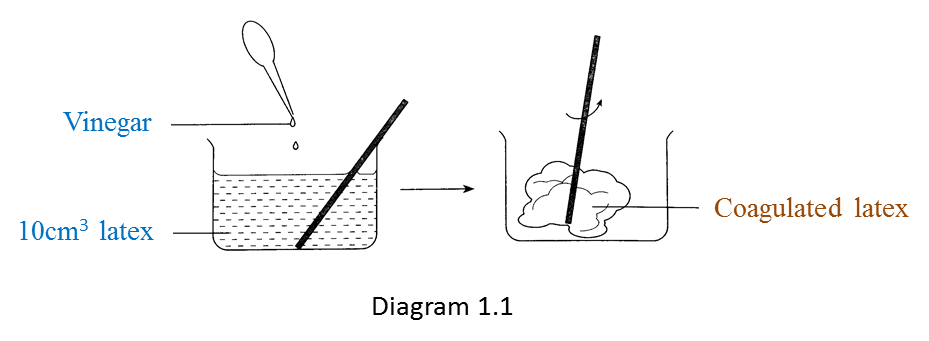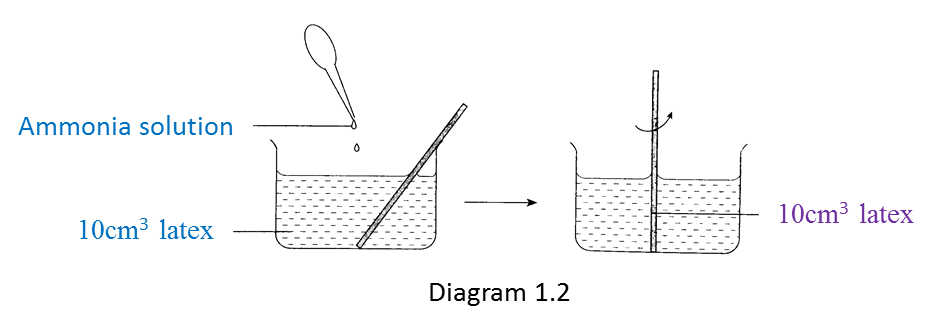(a) Write down one observation from the result of this experiment. [1 mark]

(b) State one inference based on the observation in Diagram 1.1. [1 mark]

(c) State one constant variable in this experiment. [1 mark]

(d) State one hypothesis for this experiment. [1 mark]

(e) Based on this experiment, state the operational definition for acid. [1 mark]

:
(a)
Vinegar causes the coagulation of latex but ammonia solution prevents the coagulation of latex.

(b)
When vinegar (an acid) is mixed with latex, the latex coagulates.
When ammonia solution (an alkali) is mixed with latex, the latex does not coagulate.
(any one)

(c)

1. 10 cm3 of latex
2. Results of the experiment are observed after 30 minutes
(any one)

(d)
Acid causes the coagulation of latex but alkali prevents the coagulation of latex.

(e)
Acid is a substance which causes latex to coagulate.

Question 5:
Diagram 2.1 shows the process of latex coagulation.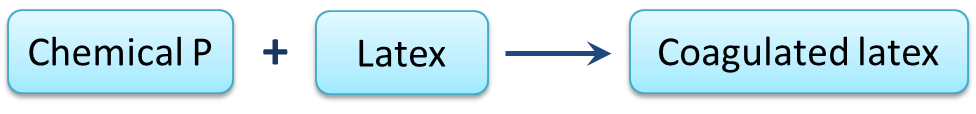Diagram 5.1

(a)(i) State one example of chemical P? [1 mark]

(ii) State one characteristic of coagulated latex in Diagram 5.1. [1 mark]

(b) Chemical P in Diagram 5.1 is replaced with chemical Q to prevent latex from coagulating.
State one example of chemical Q. [1 mark]

(c) Diagram 5.2 shows the process when natural rubber is heated with Sulphur to form rubber R.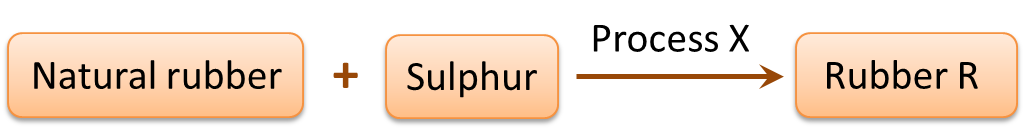Diagram 5.2

(i) Name process X. [1 mark]
(ii) Name rubber R. [1 mark]

(d) Mark (√) the object which is made of rubber R. [1 mark](a)(i)
Methanoic acid

(a)(ii)
Elastic

(b)
Ammonia solution

(c)(i)
Vulcanization of rubber

(c)(ii)
Vulcanized rubber

(d)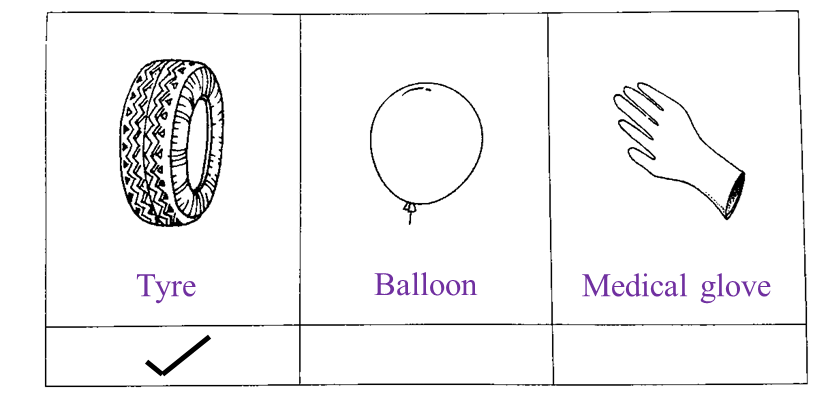### 1 thought on “Polymer – Part 3 (Structured Questions)”

1.the information are really useful for me.Thank you so much for the information